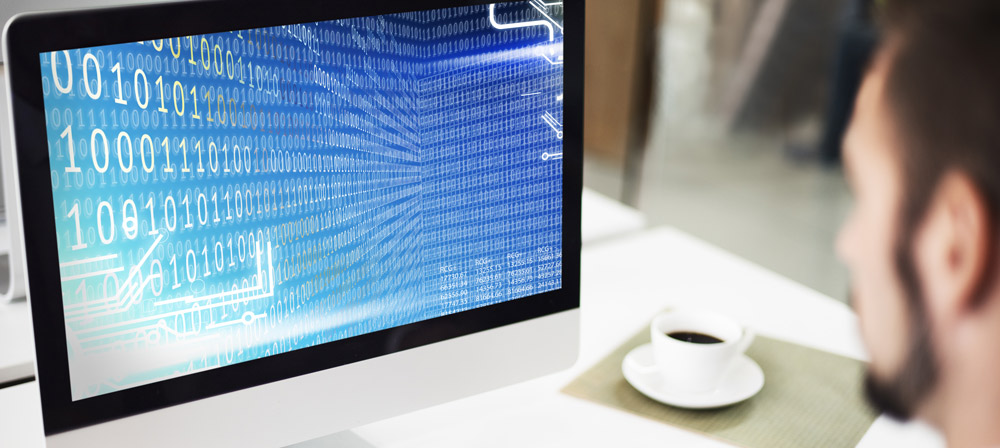#### Are You Looking for Developers to Hire?Have a Look at Our Bench Strength# How to Convert Decimal Numbers to Binary?

By Arun KumarThe process of converting decimal numbers to binary involves dividing the decimal number repeatedly by 2, recording the remainders, and then reversing the sequence of remainders to obtain the binary equivalent. Here's the step-by-step process:

1. Divide the decimal number by 2.
2. Write down the remainder.
3. Repeat steps 1 and 2 until the quotient becomes zero.
4. The binary equivalent is the sequence of remainders in reverse order.

For example, to convert the decimal number 19 to binary, the process would be:

19 ÷ 2 = 9 with a remainder of 1

9 ÷ 2 = 4 with a remainder of 1

4 ÷ 2 = 2 with a remainder of 0

2 ÷ 2 = 1 with a remainder of 0

1 ÷ 2 = 0 with a remainder of 1

The binary equivalent of 19 is 10011.